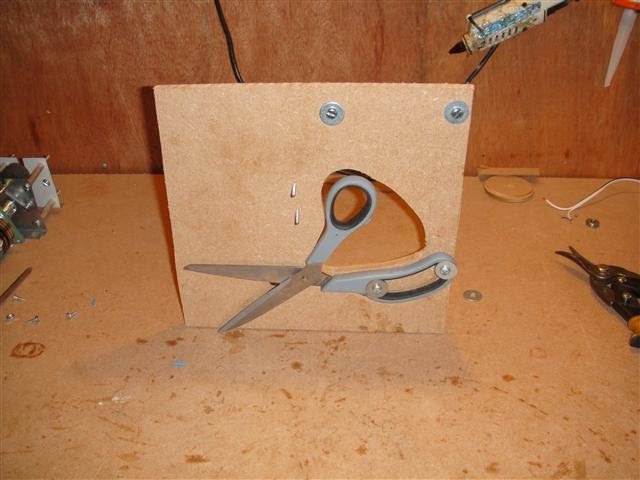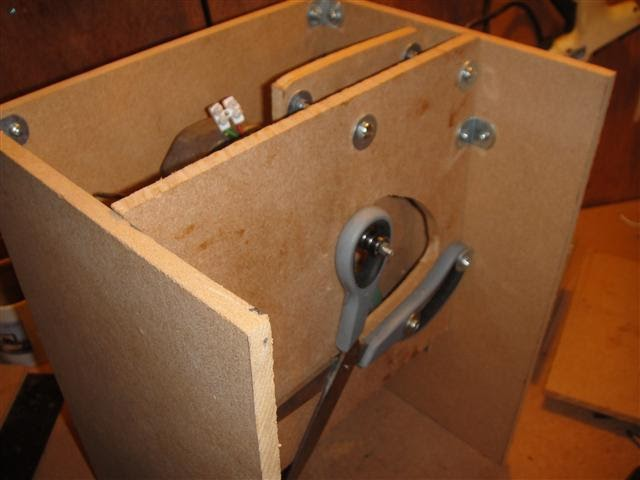Raspberry Pi‎ > ‎

### Tubing cutter

Automatic tubing cutter using Raspberry Pi

This machine automatically cuts tubing down to a certain length. It's controlled by a Raspberry Pi microcomputer, the size and quantity required are input into the machine using a USB numpad, the machine then cuts the tubing to the required length.

The cutter is a pair of strong scissors driven by a windscreen wiper motor. The wire is fed through the scissors using a stepper motor.

Below is the first part I made, the scissors attached to a piece of 9 mm MDF.This is the wire feeding mechanism. It's two rubber wheels, one of which is spring loaded. The rubber wheels are sandwiched between two pieces of steel taken from an old PC case. The rubber wheels came out of a printer.

I used an old windscreen wiper motor to drive the cutter. This motor is perfect as it already goes back and forth, via a mechanical linkage inside. This means that I don't need a H-Bridge to drive it, just a relay. It also has limit switches built in, these are fed into the RPi, so that the program knows where the cutter is (approximately).

The windscreen wiper motor fixed into place. The arm on the motor has a bolt with ball bearings on the end, this fits into the scissor handles.

Another shot of the stepper and windscreen motor.

Front of the machine, showing how the motor meets with the scissors.On top of the machined there are the following

1. USB Numpad for input of values.
2. Green Reset button (should have made it red.... never mind).
3. LED 1 indicates the program is waiting for length to be input.
4. LED 2 indicates the program is waiting for quantity to be input.
5. Big green toggle switch for on off.

The control circuit and Raspberry Pi :

The motor is driven from a large automotive relay, the relay is drive by a darlington transistor.
The stepper motor is also driven from 4 darling transistors.

I wanted the raspberry pi to run off the same power supply as the machine, but I couldn't get it to word, as the powersupply I have is too weak. As soon as the motor turns on the voltage drops right down. I may try and fix this with some large capacitors, but for now I'm running the RPi on a separate power supply.

I used some python code to control everything (could definitely be improved, but it works)

#### Text Box

import RPi.GPIO as GPIO
import time

GPIO.setup (23, GPIO.OUT)
GPIO.setup (18, GPIO.OUT)
GPIO.setup (19, GPIO.OUT)
GPIO.setup (8, GPIO.OUT)
GPIO.setup (7, GPIO.OUT)
GPIO.setup (5, GPIO.OUT)
GPIO.setup (10, GPIO.OUT)

GPIO.setup (13, GPIO.IN)
GPIO.setup (26, GPIO.IN)

motorpos = 0
length = 0
length2 = 0
quant = 0
waittime = 0.005
quit = GPIO.input(26)

while quit == True:
GPIO.output(18, True)
GPIO.output(19, True)
time.sleep(0.05)
GPIO.output(18, False)
GPIO.output(19, False)
time.sleep(0.05)
quit = GPIO.input(26)

GPIO.output(5, False)
GPIO.output(7, False)
GPIO.output(8, False)
GPIO.output(10, False)
GPIO.output(23, False)

quit = GPIO.input(26)

while quit == True:
GPIO.output(10, False)
GPIO.output(5, True)
GPIO.output(7, True)
time.sleep(waittime)
GPIO.output(5, False)
GPIO.output(7, True)
GPIO.output(8, True)
time.sleep(waittime)
GPIO.output(7, False)
GPIO.output(8, True)
GPIO.output(10, True)
time.sleep(waittime)
GPIO.output(8, False)
GPIO.output(10, True)
GPIO.output(5, True)
time.sleep(waittime)
quit = GPIO.input(26)

GPIO.output(23, True)
time.sleep(0.5)
motorpos = GPIO.input(13)
while motorpos == False:
motorpos = GPIO.input(13)
GPIO.output(23, False)

while True:
quancount = 0
length2 = 0

GPIO.output(19, True)
length = int(raw_input('Enter length : '))
GPIO.output(19, False)
GPIO.output(18, True)
quant = int(raw_input('Enter quantity: '))
GPIO.output(18, False)

while quancount < quant:
while length2 < length:
GPIO.output(10, False)
GPIO.output(5, True)
GPIO.output(7, True)
time.sleep(waittime)
GPIO.output(5, False)
GPIO.output(7, True)
GPIO.output(8, True)
time.sleep(waittime)
GPIO.output(7, False)
GPIO.output(8, True)
GPIO.output(10, True)
time.sleep(waittime)
GPIO.output(8, False)
GPIO.output(10, True)
GPIO.output(5, True)
time.sleep(waittime)
length2 += 1

GPIO.output(5, False)
GPIO.output(7, False)
GPIO.output(8, False)
GPIO.output(10, False)
GPIO.output(23, True)
time.sleep(0.5)
motorpos = GPIO.input(13)
while motorpos == False:
motorpos = GPIO.input(13)
GPIO.output(23, False)
quancount +=1
length2 = 0
quit = GPIO.input(26)
if quit == False:
quancount = quant

To get the program to autorun I modified the rc.local file to run my python script at start up. I also had to make the python script executable.

Raspberry Pi is a trademark of the Raspberry Pi Foundation